# [Java] [Processing x Java] How to use loop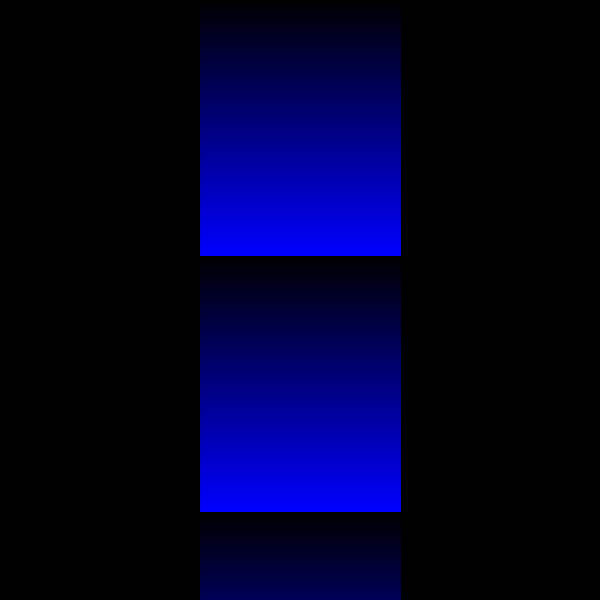1. What to use the loop for 1.for loop
2. Example of program using loop

# 0. What to use the loop for

Used for combining iterative processing. The program below is a program for efficiently obtaining the sum of numbers from 1 to 10.

#### `loop00.java`

``````
// Variable sum is the sum you want
int sum = 0;

// Add i by 1 from 1 to 10.
for(int i = 1;i <11;i++){
//Sum (new) = sum (old) + i
sum = sum + i;
}

//Display the sum on the console.
println(sum);
``````
``````55
``````
i sum sum + i
1 1 0 + 1
2 3 1 + 2
3 6 3 + 3
4 10 6 + 4
5 15 10 + 5
6 21 15 + 6
7 28 21 + 7
8 36 28 + 8
9 45 36 + 9
10 55 45 + 10

Point: In order to use loops, we have to find a common part (property/law) among similar repetitive actions! So you have to figure out which part to repeat.

# 1.for loop

## for loop

A for loop is one of the means to create a loop. It’s the same as doing a while loop, but this one is more compact and easier to understand, so I’ll introduce it here.

####◯ A program that repeatedly draws lines

#### `loop01.java`

``````
// Determine the size of the screen.
size(500,250);
// Place lines every 20 px.
for(int i = 0;i <width;i += 20){
line(i,0,i,height);
}
``````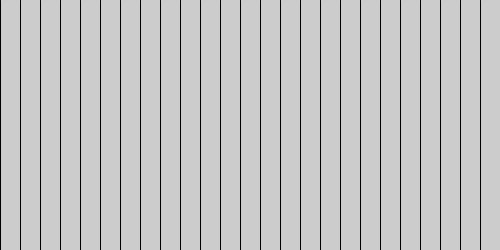Point: A program that draws a straight line drawn from (i,0) to (i,height) every 20px within the range of i <width.

#### ○ For loop execution order (When compared with this program…)

what is the value of i? : 0! ↓ Does the value of i (=0) satisfy i <width? : True! ↓ Execution of processing ↓ i += 20! ↓ what is the value of i? : 20! : : what is the value of i? : 500! ↓ Does the value of i (=500) satisfy i <width? : False! (End of loop)

Point: i += 20 is an abbreviation for i = i + 20.

#### ○ for loop image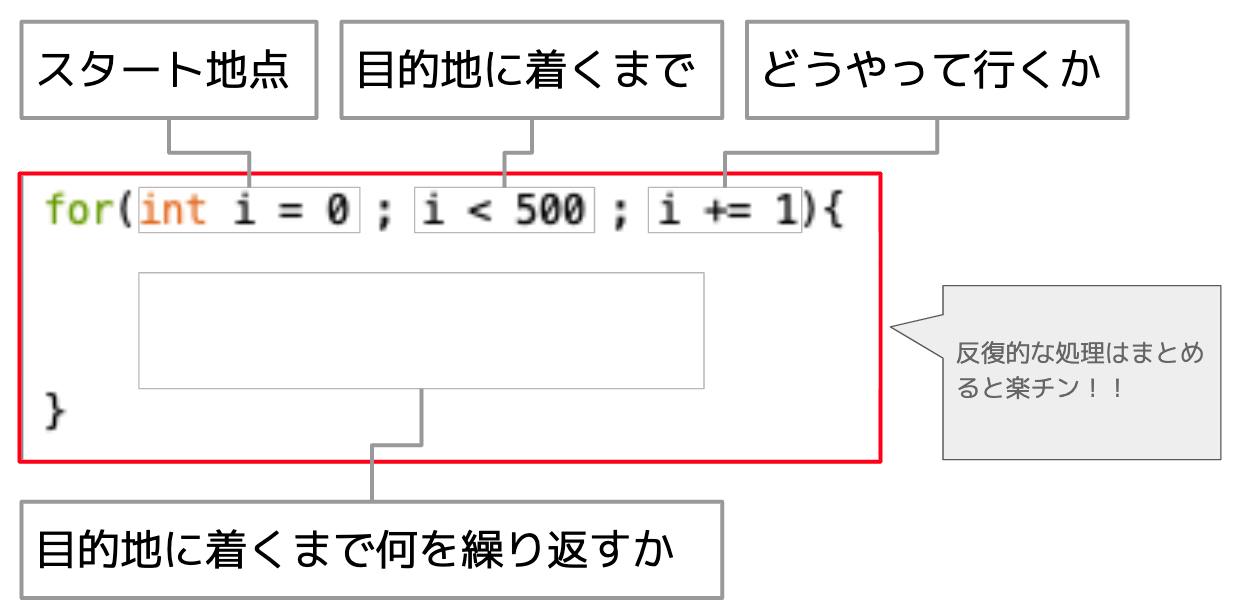Point: It may be close to that feeling of choosing a snack for a field trip so that it will fit in 300 yen…

# 2. Example of program using for loop

####◯ A program that gradually shifts the lines

#### `variable02.java`

``````
// Determine screen size
size(500,500);
// decide the background color
background(0);

//Increase i by 10 while satisfying i <width.
for(int i = 0;i <width;i += 10){
// decide the line color
stroke(53,183,193);
// Draw a line from (0,i) to (i,height)
line(0,i,i,height);
}
``````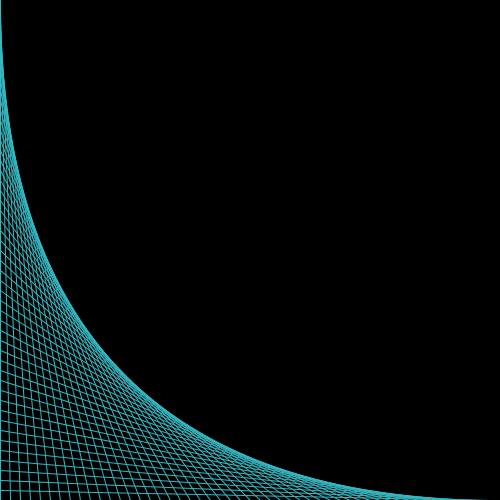Point: Processing coordinates ![Screenshot 2020-06-30 20.42.39.png](https://qiita-image-store.s3.ap-northeast-1.amazonaws.com/0/654004/c5b49bbe-01f7-0292-8efa-(1bfd7bb9fee1.png) The feature is that the value of y increases as it goes to the bottom of the screen.

####◯ A program that draws lines randomly

#### `variable03.java`

``````
// Determine screen size
size(500,500);
// decide the background color
background(0);

//Increase i by 1 while satisfying i <width. (Repeat 500 times)
for(int i = 0;i <width;i += 1){
// decide the line color
stroke(#640915);
// draw a line from a random point to a random point
line(random(500),random(500),random(500),random(500));
}
``````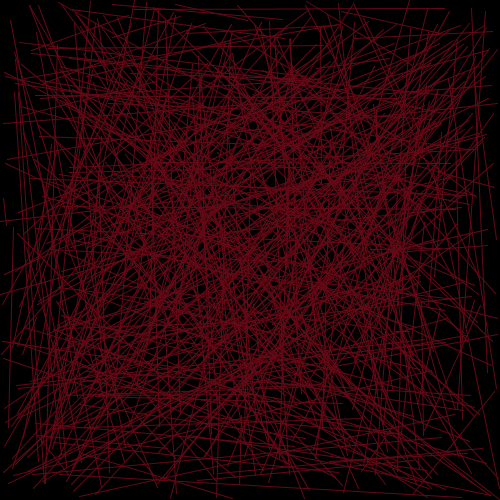Point: random(min,max); Returns (prints) a random value in the range min to max. min can be omitted.

Point: Until i becomes 0,1,2,….498,499, that is, the loop is repeated 500 times

Point: There are other methods besides choosing the color (R, G, B). From the menu bar, select [Tools] → [Select Color] → select your favorite color

####◯ Loops and animations

It is effective to use draw() to create an animation.

#### `variable03.java`

``````
//Define the variable s that represents the size of the circle
float s = 0;

// repeat only once
void setup(){
size(600,600);
// Default is 60 frames per second
frameRate(10);
background(0);
}

//infinite loop
void draw(){
// Increase the size by 1
s += 1;
//Specify the color
fill(random(255),0,255,50);
noStroke();
// Place circles at random places
ellipse(random(width),random(height),s,s);

// if the size is greater than 45 it will be executed
if (s> 45) {
// Reset size to 0
s = 0;
}

}
``````

![Screenshot 2020-07-02 8.36.00.png](https://qiita-image-store.s3.ap-northeast-1.amazonaws.com/0/654004/ffae9379-6270-36b7-eb65-(14c2b1a7852d.png)